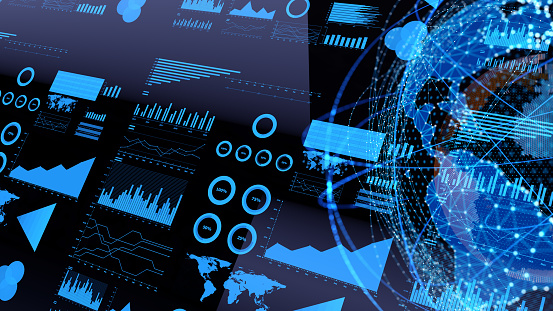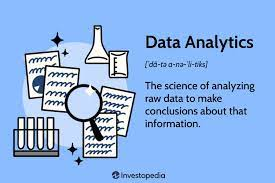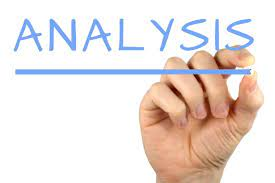Forecasting is the process of creating a forecast by using statistical methods and models to analyze current trends and develop predictions of future events. A forecasting model can be used to analyze data from past events to develop a prediction of future events. The purpose of forecasting is to help decision-makers understand the future and make informed decisions.

There are a number of different types of forecasting models, including linear regression models, time series models, and neural networks. Linear regression models are used to predict the future behavior of a dependent variable (such as sales) based on a number of independent variables (such as prices, production levels, and customer demand).

Forecasting can be used by businesses and governments to understand trends and make informed decisions. The most common forecasting methods are trend analysis and regression analysis.

Trend analysis is used to identify long-term patterns in data. It can be used to predict future events by extrapolating from past data.

Regression analysis is used to identify relationships between variables. It can be used to project future events by predicting how one variable will change as a result of changes in another.

Methods of Forecasting

There are a variety of ways to forcast weather conditions. Some of the most common include using computer models, analyzing data from weather stations, and consulting with meteorologists.

Computer models are used to simulate the weather, and can be used to provide forecasts for a particular area for a period of up to 10 days. The accuracy of computer models is determined by the quality of the data used to create them, and by the accuracy of the algorithms used to simulate the weather.

Data from weather stations can be used to provide information about temperature, humidity, barometric pressure, and wind speed.

Forecasting Models

Forecasting models are mathematical models that are used to predict future events. There are a number of different types of forecasting models, but all of them share a common goal- to generate a prediction about the future based on past events.

One of the most common types of forecasting models is the linear regression model and regression model with a time lag.

A regression model with a time lag works by predicting future events based on the relationship between past

Linear regression model

There are a number of different types of linear regression models that can be used to predict the outcome of a given experiment or analysis. Some of the most common types of linear regression models are:

• To predict the outcome of an experiment or analysis by estimating the relationship between a set of input variables and an output variable.
• To predict the outcome of an experiment or analysis by estimating the change in the output variable as a result of a change in the set of input variables.

Time series model of forecasting

In recent years, time series models have become increasingly popular in forecasting. A time series model is a model that uses time as a variable. This can be a useful tool when you want to understand how a particular event or trend affects subsequent events or trends.

There are many types of time series models, but the most common is the linear regression model. In this model, you predict future values of a variable by predicting past values. You can use this model to understand how changes in a variable affect future events or trends.

Another type of time series model is the nonlinear regression model. This model uses a more complex mathematical approach to predict future values.## AWOL & Ajaokuta Steel Mill – April 21 to 23.

in